# NCERT Solutions for Class 6 Maths Chapter 6: Integers Exercise 6.1

## NCERT Solutions for Class 6 Maths Chapter 6 Exercise 6.1

In this page we have NCERT Solutions for Class 6 Maths Chapter 6: Integers Exercise 6.1. Hope you like them and do not forget to like , social share and comment at the end of the page.
• The collection of numbers..., - 4, - 3, - 2, - 1, 0, 1, 2, 3, 4, ... is called integers.
• - 1, - 2, - 3, - 4, ... called negative numbers are negative integers
• 1, 2, 3, 4, ... called positive numbers are the positive integers
Question 1
Write opposites of the following:
(a) Increase in weight
(b) 30 km north
(c) 326 BC
(d) Loss of Rs 700
(e) 100 m above sea level
a)      Decrease in weight
b)      30 km south
c)      326 A.D.
d)     Gain of Rs. 700
e)      100 m below sea level

Question 2
Represent the following numbers as integers with appropriate signs.
(a) An aero plane is flying at a height two thousand metre above the ground.
(b) A submarine is moving at a depth, eight hundred metre below the sea level.
(c) A deposit of rupees two hundred.
(d) Withdrawal of rupees seven hundred.
a)      +2000 m
b)      –800 m
c)      + 200
d)     –700
Question 3
Represent the following numbers on a number line:
(a) + 5
(b) − 10
(c) + 8
(d) − 1
(e) – 6
All the number are shown on number line below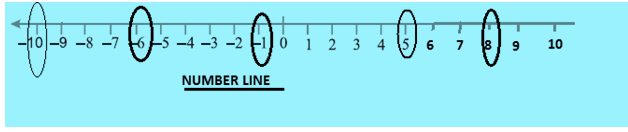Question 4
Adjacent figure is a vertical number line, representing integers. Observe it and locate the following points:
(a) If point D is + 8, then which point is − 8?
(b) Is point G a negative integer or a positive integer?
(c) Write integers for points B and E.
(d) Which point marked on this number line has the least value?
(e) Arrange all the points in decreasing order of value.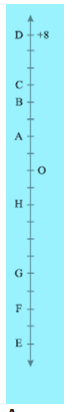Let us complete the number line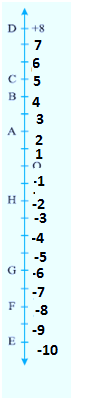We can easily find the answer for the questions from Number line
 a) F is -8 position b) G lies below 0 and it is a negative integer c) B is 4 position and E is -10 position  d) E has the least value of -10  e) D > C > B > A > O> H> G > F> E

Question 5
Following is the list of temperatures of five places in India on a particular day of the year.
 Place Temperature Siachin 10°C below 0°C …… Shimla 2°C below 0°C …… Ahmedabad 30°C above 0°C …… Delhi 20°C above 0°C …… Srinagar 5°C below 0°C ……
(a) Write the temperatures of these places in the form of integers in the blank column.
(b) Following is the number line representing the temperature in degree Celsius.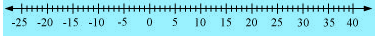Plot the name of the city against its temperature.
(c) Which is the coolest place?
(d) Write the names of the places where temperatures are above 10°C.
 Place Temperature Integer form Siachin 10°C below 0°C -10°C Shimla 2°C below 0°C -2°C Ahmedabad 30°C above 0°C 30°C Delhi 20°C above 0°C 20°C Srinagar 5°C below 0°C -5°C
b)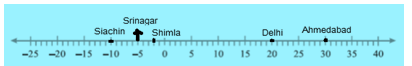c) The coolest place has the lowest temperature. From the number line above, we see that the lowest temperature is -10°C (Siachin)
So, Siachin is the coolest place.
d)     Ahmedabad and Delhi has temperatures more than 10°C.

Question 6
In each of the following pairs, which number is to the right of the other on the number line?
(a) 2, 9
(b) − 3, − 8
(c) 0, − 1
(d) − 11, 10
(e) − 6, 6
(f) 1, − 100
We know that in Number line, the larger number is always to the right of the other on a number line.
To find larger number, we can follow these steps
1) When comparing two positive integers, here are the tips
 If two numbers have unequal number of digits, then the number with the greater number of digits is greater. If two numbers have equal number of digits then, the number with greater valued digit on the extreme left is greater. If the digits on extreme left of the numbers are equal, then the digits to the right of the extreme left digits are compared and so on.
2)  When comparing two negative integers, we can remove the sign and compare them as positive integer given above, the smallest of them would be the greater when we put the sign back
3)We comparing two different sign numbers, the number with positive sign is larger
(a)      9
(b)      -3
(c)      0
(d)     10
(e)      6
(f)       1
Question 7
Write all the integers between the given pairs (write them in the increasing order.)
(a) 0 and − 7
(b) − 4 and 4
(c) − 8 and − 15
(d) − 30 and − 23
(a)      -6, -5, -4, -3, -2, -1
(b)      -3, -2, -1, 0, 1, 2, 3
(c)      -14, -13, -12, -11, -10, -9
(d)     -29, -28, -27, -26, -25, -24

Question 8
(a) Write four negative integers greater than − 20.
(b) Write four negative integers less than − 10.
a)      –1, –2, –3, –4
b)      –15, –20, –25, –30
Question 9
For the following statements, write True (T) or False (F). If the statement is false, correct the statement.
(a) − 8 is to the right of − 10 on a number line.
(b) − 100 is to the right of − 50 on a number line.
(c) Smallest negative integer is − 1
(d) − 26 is greater than − 25.
a) True
b) False
c) False
d) False

Question 10
Draw a number line and answer the following:
(a) Which number will we reach if we move 4 numbers to the right of − 2.
(b) Which number will we reach if we move 5 numbers to the left of 1.
(c) If we are at − 8 on the number line, in which direction should we move to reach − 13?
(d) If we are at − 6 on the number line, in which direction should we move to reach − 1?

(a)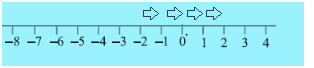So we reach number 2
(b)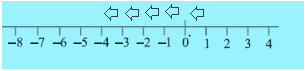So we reach number -4
(c) We should move in left direction to reach -13
(d) We have to move in right direction to reach -1

## Try these NCERT Book questions

Question 1. Write the following numbers with appropriate signs :
(a) 100 m below sea level.
(b) 25°C above 0°C temperature.
(c) 15°C below 0°C temperature.
(d) any five numbers less than 0
a. -100m
b. 25°C
c. -15°C
d. -1,-2,-3,-4,-5

Question 2. Compare the following pairs of numbers using > or <.
a. 0 ___ -8
b. - 1 ____ -15
c. 5 ____ -5
d. 11 ___15
e. 0 ____ 6
f. - 20 ___ 2
a. 0 > -8
b. -1 > -15
c. 5 >-5
d. 11 < 15
e. 0 < 6
f. -20 < 2

Question 3.From the above exercise, Rohini arrived at the following conclusions :
(a) Every positive integer is larger than every negative integer.
(b) Zero is less than every positive integer.
(c) Zero is larger than every negative integer.
(d) Zero is neither a negative integer nor a positive integer.
(e) Farther a number from zero on the right, larger is its value.
(f) Farther a number from zero on the left, smaller is its value.
Do you agree with her? Give examples.
a. True
b. True
c. True
d. True
e. True
f. True### Practice Question

Question 1 What is $\frac {1}{2} + \frac {3}{4}$ ?
A)$\frac {5}{4}$
B)$\frac {1}{4}$
C)$1$
D)$\frac {4}{5}$
Question 2 Pinhole camera produces an ?
A)An erect and small image
B)an Inverted and small image
C)An inverted and enlarged image
D)None of the above